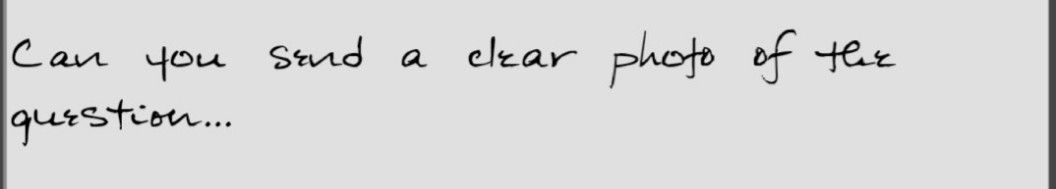Symbol
ProblemB. $111$ graph of y $1$ is shown below. Based on this graph, sketch $100$ graphs of $11n0$ following quadratic functions on the same coordinate plane. 1. y $x^{2}-2$ 2. $3$ $4.y$ $-3\left(x-5\right)^{2}$ $=3\left(x+1\right)^{2}+3$ $=3\left(x-4\right)^{2}-2$ C. Delemine the functions whose graphs are described below. Draw the graph. 1. The graph of y = 2x shifted 4 units to the left 2. The graph of y= -x shifted 3 units upward 3. The graph of y 3x shifted 2 units to the right $and$ $1$ $4n11downwa$ $-$ $-$ 4. The graph of y = $2$ shifted 1 unit to the left $0n$ $21$ units upward -5 $0$
7th-9th grade
Other
Search count: 122
SolutionQanda teacher - AskhinaStudent
okay just give me a sec:)
i can't send a new photo